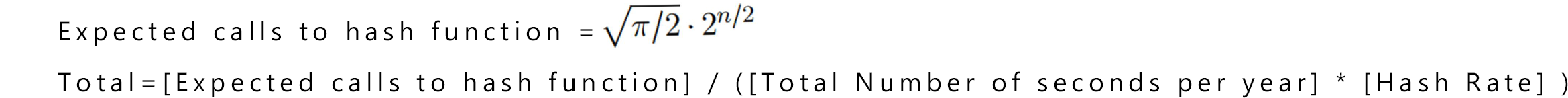Formula used to calculate number of years to find a hash collisionEnter in a hash rate (in trillions of Hashes per second):
Enter in number of bits:

Your hash rate was: 0
The number of bits to calculate was:
The calculated number of years for a collision is: inf years